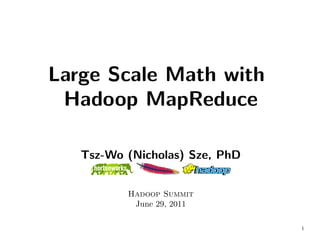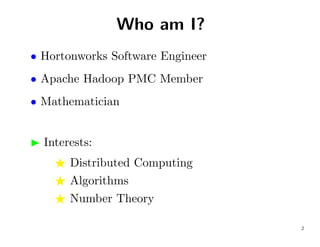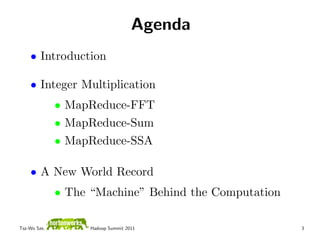Successfully reported this slideshow.

# Large Scale Math with Hadoop MapReduce

47

Share×
1 of 77
1 of 77

# Large Scale Math with Hadoop MapReduce

47

Share

Hadoop Summit 2011 presentation on Large Scale Math with Apache Hadoop MapReduce

Hadoop Summit 2011 presentation on Large Scale Math with Apache Hadoop MapReduce

## More Related Content

### Related Books

Free with a 30 day trial from Scribd

See all

### Related Audiobooks

Free with a 30 day trial from Scribd

See all

### Large Scale Math with Hadoop MapReduce

1. 1. Large Scale Math with Hadoop MapReduce Tsz-Wo (Nicholas) Sze, PhD Hadoop Summit June 29, 2011 1
2. 2. Who am I? • Hortonworks Software Engineer • Apache Hadoop PMC Member • Mathematician Interests: Distributed Computing Algorithms Number Theory 2
3. 3. Agenda • Introduction • Integer Multiplication • MapReduce-FFT • MapReduce-Sum • MapReduce-SSA • A New World Record • The “Machine” Behind the Computation Tsz-Wo Sze, Hadoop Summit 2011 3
4. 4. Agenda • Introduction • Integer Multiplication • MapReduce-FFT • MapReduce-Sum • MapReduce-SSA • A New World Record • The “Machine” Behind the Computation Tsz-Wo Sze, Hadoop Summit 2011 4
5. 5. Typical Hadoop Applications Major applications of Hadoop include • Search and crawling • Text processing • Machine learning • ... Tsz-Wo Sze, Hadoop Summit 2011 5
6. 6. Typical Hadoop Applications Major applications of Hadoop include • Search and crawling • Text processing • Machine learning • ... But not yet commonly used in scientiﬁc or mathematical applications. Why? Tsz-Wo Sze, Hadoop Summit 2011 6
7. 7. Why Not Math? No MapReduce math libraries available, and More fundamentally, MapReduce math algorithms are not well studied. Tsz-Wo Sze, Hadoop Summit 2011 7
8. 8. Existing Library Really no MapReduce Math Library? Not exactly. Tsz-Wo Sze, Hadoop Summit 2011 8
9. 9. Existing Library Really no MapReduce Math Library? Not exactly. Apache Mahout • A machine learning library. • Includes packages for matrix operations. Tsz-Wo Sze, Hadoop Summit 2011 9
10. 10. Existing Library Really no MapReduce Math Library? Not exactly. Apache Mahout • A machine learning library. • Includes packages for matrix operations. Apache Hama (Incubation) • A matrix computational package. Tsz-Wo Sze, Hadoop Summit 2011 10
11. 11. Computational Intensive Problems (1) Integer Factoring • a.k.a. breaking RSA cryptosystem Given N , e and c, compute m such that     e c ≡ m (mod N ),       where N is a product of two primes. • a 768-bit RSA modulus was factored1 in 2009 1 Kleinjung et al., Factorization of a 768-bit RSA modulus, CRYPTO 2010. Tsz-Wo Sze, Hadoop Summit 2011 11
12. 12. Computational Intensive Problems (2) Solving PDEs (Partial Diﬀerential Equations) • Fluid dynamics • Electromagnetism • Financial analysis • ... (Two-dimensional Turbulence, courtesy of Y.K. Tsang) Tsz-Wo Sze, Hadoop Summit 2011 12
13. 13. Computational Intensive Problems (3) Finding complex zeros of Riemann Zeta function ∞ 1 ζ(s) = for s ∈ C, (s) > 1 n=1 ns and then analytically continued to all s = 1. Tsz-Wo Sze, Hadoop Summit 2011 13
14. 14. Computational Intensive Problems (3) Finding complex zeros of Riemann Zeta function ∞ 1 ζ(s) = for s ∈ C, (s) > 1 n=1 ns and then analytically continued to all s = 1. • Disprove Riemann Hypothesis (RH) Then, you will get \$1,000,000 dollars2. However, RH is unlikely to be false. 2 See http://www.claymath.org/millennium/Riemann_Hypothesis/. Tsz-Wo Sze, Hadoop Summit 2011 14
15. 15. Computational Intensive Problems (3) Finding complex zeros of Riemann Zeta function ∞ 1 ζ(s) = for s ∈ C, (s) > 1 n=1 ns and then analytically continued to all s = 1. • Disprove Riemann Hypothesis (RH) Then, you will get \$1,000,000 dollars. However, RH is unlikely to be false. • More likely: Obtain more evidents which support RH. Tsz-Wo Sze, Hadoop Summit 2011 15
16. 16. Computational Intensive Problems (4) Computing π Latest world records: • Five trillion decimal digits (August 2010) by Alexander Yee & Shigeru Kondo3 3 See http://www.numberworld.org/misc_runs/pi-5t/announce_en.html Tsz-Wo Sze, Hadoop Summit 2011 16
17. 17. Computational Intensive Problems (4) Computing π Latest world records: • Five trillion decimal digits (August 2010) by Alexander Yee & Shigeru Kondo • The two quadrillionth bits (July 2010) by Tsz-Wo Sze & the Yahoo! Cloud Computing Team4 4 See http://developer.yahoo.net/blogs/hadoop/2010/09/two_quadrillionth_bit_pi.html Tsz-Wo Sze, Hadoop Summit 2011 17
18. 18. Missing Functionalities Fast Fourier Transform (FFT) – the basic rountine behind many algorithms. Arbitrary Precision Arithmetic Integer functions Floating-point functions Complex functions ... Tsz-Wo Sze, Hadoop Summit 2011 18
19. 19. Agenda • Introduction • Integer Multiplication • MapReduce-FFT • MapReduce-Sum • MapReduce-SSA • A New World Record • The “Machine” Behind the Computation Tsz-Wo Sze, Hadoop Summit 2011 19
20. 20. Why Integer Multiplication? There exist fast algorithms. Many applications • Division • Logarithm • Trigonometric functions • ... Tsz-Wo Sze, Hadoop Summit 2011 20
21. 21. Prerequisite of Algorithms D.J. Bernstein, Fast multiplication and its applications, ANTS 2008. Tsz-Wo Sze, Hadoop Summit 2011 21
22. 22. Integer Multiplication Algorithms Na¨ O(N 2) ıve, Karatsuba, O(N log2 3) = O(N 1.585) Toom-Cook, O(N log(2D−1)/ log D ) If D = 3, then O(N log 5/ log 3) = O(N 1.465) FFT-based algorithms O(N log N · · · ) Tsz-Wo Sze, Hadoop Summit 2011 22
23. 23. FFT-based Algorithms Basic FFT, O(N log N log log N log log log N · · · ) Sch¨nhage-Strassen, O(N log N log log N ) o Nussbaumer, O(N log N log log N ) log∗ N F¨rer, O(N (log N )2 u ) log∗ N De-Kurur-Saha-Saptharishi, O(N (log N )2 ) Tsz-Wo Sze, Hadoop Summit 2011 23
24. 24. Convolution By the convolution theorem, a × b = dft−1(dft(a) ∗ dft(b)), where × denotes the convolution operator , ∗ denotes componentwise multiplication, dft( · ) denotes discrete Fourier transform. Tsz-Wo Sze, Hadoop Summit 2011 24
25. 25. Sch¨nhage-Strassen Algorithm o (SSA) Represent integers as polynomials. Then, com- pute convolution with DFTs modulo an integer5. 5 It has the form 2n + 1 and is called the Sch¨nhage-Strassen modulas. o Tsz-Wo Sze, Hadoop Summit 2011 25
26. 26. SSA Steps Step 1: two DFTs, def ˆ def dft(b); ˆ a = dft(a) and b= Step 2: componentwise multiplication, def ˆ ˆ p = a ∗ b; ˆ Step 3: a DFT inverse, −1 p = dft (ˆ ); p Step 4: normalization. Tsz-Wo Sze, Hadoop Summit 2011 26
27. 27. Calculating DFTs DFT can be calculated by a family of algorithms called Fast Fourier Transform (FFT). Tsz-Wo Sze, Hadoop Summit 2011 27
28. 28. FFT Family Recursive-FFT Parallel-FFT Cooley-Tukey (decimation-in-time) Gentleman-Sande (decimation-in-frequency) Danielson-Lanczos Ping-pong FFT ... Tsz-Wo Sze, Hadoop Summit 2011 28
29. 29. Data Model (1) Need a data model which allows accessing terabit integers eﬃciently. An integer x is represented as a D-dimensional tuple x = (xD−1, xD−2, . . . , x0). Tsz-Wo Sze, Hadoop Summit 2011 29
30. 30. Data Model (2) Write D = IJ. where I and J are powers of two. Deﬁne J-dimensional tuples (i) def x = (x(J−1)I+i, x(J−2)I+i, . . . , xi) for 0 ≤ i < I. Tsz-Wo Sze, Hadoop Summit 2011 30
31. 31. Data Model (3) Then,     x(0) x(J−1)I x(J−2)I . . . x0  (1)    x   x(J−1)I+1 x(J−2)I+1 . . . x1    . = . . ... .   .   . . .  x(I−1) x(J−1)I+(I−1) x(J−2)I+(I−1) . . . xI−1 We call it the (I, J)-format of x. Tsz-Wo Sze, Hadoop Summit 2011 31
32. 32. Data Model (4) Each x(i) is a sequence of J records. Each record is a key-value pair. Record # <Key, Value> 0 < i, xi > 1 < J + i, xJ+i > . . . . J −1 < (J − 1)I + i, x(J−1)I+i > Tsz-Wo Sze, Hadoop Summit 2011 32
33. 33. Data Model (5) Thus, an integer is stored as I SequenceFiles in HDFS, each SequenceFile contains J records. Tsz-Wo Sze, Hadoop Summit 2011 33
34. 34. Parallel-FFT Steps Step 1: I inner DFTs with J-point, a(i) = dft(a(i)); Step 2: componentwise shifting, def zjI+i = ζ ij a(i)j ; Step 3: transposition, [j] def z = (zjI+(I−1), zjI+(I−2), . . . , zjI ); Step 4: J outer DFTs with I-point, [j] def z = dft(z[j]). Tsz-Wo Sze, Hadoop Summit 2011 34
35. 35. MapReduce Model Input Map1 Map2 Map3 Map4 Shuffle Reduce1 Reduce2 Reduce3 Reduce4 Output Tsz-Wo Sze, Hadoop Summit 2011 35
36. 36. MapReduce-FFT Input Inner FFT1 Inner FFT2 Inner FFT3 Inner FFT4 Transposition (by shuffle) Outer FFT1 Outer FFT2 Outer FFT3 Outer FFT4 Output Tsz-Wo Sze, Hadoop Summit 2011 36
37. 37. Data Locality The FFT transposition, which is traditionally dif- ﬁcult in preserving locality, becomes trivial in MapReduce. Tsz-Wo Sze, Hadoop Summit 2011 37
38. 38. MapReduce-FFT (1) Map function: (k1, v1) −→ list k2, v2 Algorithm 1 (Forward FFT, Mapper). (f.m.1) read key i, value a(i); (f.m.2) calculate a J-point DFT; (f.m.3) componentwise multiply; (f.m.4) for 0 ≤ j < J, emit key j, value (i, zjI+i). Tsz-Wo Sze, Hadoop Summit 2011 38
39. 39. MapReduce-FFT (2) Reduce function: (k2, list v2 ) −→ list k3, v3 . Algorithm 2 (Forward FFT, Reducer). (f.r.1) receive key j, list [(i, zjI+i)]0≤i<I ; (f.r.2) calculate an I-point DFT; (f.r.3) write key j, value z[j]. Tsz-Wo Sze, Hadoop Summit 2011 39
40. 40. Normalization Normalization can be viewed as a summation of three integers. Tsz-Wo Sze, Hadoop Summit 2011 40
41. 41. Summation Integer summation can be done by (1) componen- twise summation, (2) carry evaluation and then (3) parallel carrying. Tsz-Wo Sze, Hadoop Summit 2011 41
42. 42. MapReduce Model Input Map1 Map2 Map3 Map4 Shuffle Reduce1 Reduce2 Reduce3 Reduce4 Output Tsz-Wo Sze, Hadoop Summit 2011 42
43. 43. MapReduce-Sum Input Summation1 Summation2 Summation3 Summation4 Carry Evaluation (modified shuffle) Carrying1 Carrying2 Carrying3 Carrying4 Output Tsz-Wo Sze, Hadoop Summit 2011 43
44. 44. Job 1: Componwise Summation Input Summation1 Summation2 Summation3 Summation4 Output A map-only job. Tsz-Wo Sze, Hadoop Summit 2011 44
45. 45. Job 2: Carrying Input Carry Evaluation Carrying1 Carrying2 Carrying3 Carrying4 Output Tsz-Wo Sze, Hadoop Summit 2011 45
46. 46. MapReduce-SSA two concurrent forward FFT jobs; a backward FFT job with componentwise multiplication and splitting ; a componentwise summation map-only job; a carrying job6. 6 It is possible to combine the last two jobs if we modify the shuﬄe process in MapReduce [.next]. Tsz-Wo Sze, Hadoop Summit 2011 46
47. 47. Prototype Implementation DistMpMult – distributed multi-precision multiplication DistFft – distributed FFT DistCompSum – distributed componentwise summation DistCarrying – distributed carrying Open source – available at https://issues.apache.org/jira/browse/MAPREDUCE-2471 Tsz-Wo Sze, Hadoop Summit 2011 47
48. 48. Cluster Conﬁguration A shared cluster: Apache Hadoop 0.20 1350 nodes 6 GB memory per node 2 map tasks & 1 reduce task per node Imposed a limitation on the aggregated memory usage of individual jobs. Tsz-Wo Sze, Hadoop Summit 2011 48
49. 49. Running Time Actual running time for 236 ≤ N ≤ 240. 11.5 t is the elapsed time in seconds 11 10.5 10 9.5 log(t) 9 8.5 8 7.5 7 32 33 34 35 36 37 38 39 40 log(N) Tsz-Wo Sze, Hadoop Summit 2011 49
50. 50. Agenda • Introduction • Integer Multiplication • MapReduce-FFT • MapReduce-Sum • MapReduce-SSA • A New World Record • The “Machine” Behind the Computation Tsz-Wo Sze, Hadoop Summit 2011 50
51. 51. What is π? π is a mathematical constant such that, for any circle, circumference C π= = . diameter d Tsz-Wo Sze, Hadoop Summit 2011 51
52. 52. What is π? π is a mathematical constant such that, for any circle, circumference C π= = . diameter d We have π = 3.244 Tsz-Wo Sze, Hadoop Summit 2011 52
53. 53. What is π? π is a mathematical constant such that, for any circle, circumference C π= = . diameter d We have π = 3.244 (in hexadecimal ) Tsz-Wo Sze, Hadoop Summit 2011 53
54. 54. Decimal, Hexadecimal & Binary Representing π in diﬀerent bases π = 3.1415926535 8979323846 2643383279 ... = 3.243F6A88 85A308D3 13198A2E ... = 11.00100100 00111111 01101010 ... Bit position is counted after the radix point. e.g., the eight bits starting at the ninth bit position are 00111111 in binary or 3F in hexadecimal. Tsz-Wo Sze, Hadoop Summit 2011 54
55. 55. A New World Record Yahoo! Cloud Computing (July 2010) • Machines: Idle slices of 1000-node clusters Each node has two quad-core 1.8-2.5 GHz CPUs • Duration: 23 days • CPU time: 503 years • Veriﬁcation: 582 years CPU time Tsz-Wo Sze, Hadoop Summit 2011 55
56. 56. A New World Record Bit values (in hexadecimal) 0E6C1294 AED40403 F56D2D76 4026265B CA98511D 0FCFFAA1 0F4D28B1 BB5392B8 Tsz-Wo Sze, Hadoop Summit 2011 56
57. 57. A New World Record Bit values (in hexadecimal) 0E6C1294 AED40403 F56D2D76 4026265B CA98511D 0FCFFAA1 0F4D28B1 BB5392B8 (256 bits) The ﬁrst bit position: 1,999,999,999,999,997 (= 2 · 1015 − 3) The last bit position: 2,000,000,000,000,252 (= 2·1015 +252) The two quadrillionth (2 · 1015th) bit is 0. Tsz-Wo Sze, Hadoop Summit 2011 57
58. 58. BBC News (16 Sep 2010) Pi record smashed as team ﬁnds two-quadrillionth digit http://www.bbc.co.uk/news/technology-11313194 Tsz-Wo Sze, Hadoop Summit 2011 58
59. 59. NewScientist (17 Sep 2010) New pi record exploits Yahoo’s computers http://www.newscientist.com/article/dn19465-new-pi-record-exploits-yahoos-com html Tsz-Wo Sze, Hadoop Summit 2011 59
60. 60. Other News Coverage New Pi Record Exploits Yahoo’s Computers http://cacm.acm.org/news/99207-new-pi-record-exploits-yahoos-computers The Yahoo! boﬃn scores pi’s two quadrillionth bit http://www.theregister.co.uk/2010/09/16/pi_record_at_yahoo Pi calculation more than doubles old record http://www.radionz.co.nz/news/world/57128/pi-calculation-more-than-doubles-ol Hadoop used to calculate Pi’s two quadrillionth bit http://www.zdnet.co.uk/blogs/mapping-babel-10017967/hadoop-used-to-calculate- Tsz-Wo Sze, Hadoop Summit 2011 60
61. 61. Yahoo! researcher breaks Pi record in ﬁnding the two-quadrillionth digit http://www.engadget.com/2010/09/17/yahoo-researcher-breaks-pi-record-in-findi Nicholas Sze of Yahoo Finds Two-Quadrillionth Digit of Pi http://science.slashdot.org/story/10/09/16/2155227/Nicholas-Sze-of-Yahoo-Find The 2,000,000,000,000,000th digit of the mathemat- ical constant pi discovered http://news.gather.com/viewArticle.action?articleId=281474978525563 Researcher Shatters Pi Record by Finding Two-Quadrillionth Digit http://www.maximumpc.com/article/news/researcher_shatters_pi_record_finding_ two-quadrillionth_digit Tsz-Wo Sze, Hadoop Summit 2011 61
62. 62. A bigger slice of pi http://radar.oreilly.com/2010/09/strata-week-grabbing-a-slice.html 2 Quadrillionth digit of PI is found: Scientist celebration in worldwide Pandemonium http://engforum.pravda.ru/showthread.php?296242-2-Quadrillionth-digit-of-PI-i And the number is...0 http://www.hexus.net/content/item.php?item=26505 Pi Record Smashed as Team Finds Two- Quadrillionth Digit http://hardocp.com/news/2010/09/16/pi_record_smashed_as_team_finds_twoquadril digit Tsz-Wo Sze, Hadoop Summit 2011 62
63. 63. Yahoo Engineer Calculates Two Quadrillionth Bit Of Pi http://www.webpronews.com/topnews/2010/09/17/yahoo-engineer-calculates-two-qu A Cloud Computing Milestone: Yahoo! Reaches the 2 Quadrillionth Bit of Pi http://www.readwriteweb.com/cloud/2010/09/a-cloud-computing-milestone-ya. php Yahoo researcher Nicolas Sze determines the 2,000,000,000,000,000th digit of the mathematical con- stant pi http://www.thaindian.com/newsportal/sci-tech/yahoo-researcher-nicolas-sze-det 100430278.html ... Tsz-Wo Sze, Hadoop Summit 2011 63
64. 64. Computing π How to compute the nth bits of π? Tsz-Wo Sze, Hadoop Summit 2011 64
65. 65. Computing π How to compute the nth bits of π? Let’s ignore this question in this talk ... and focus on: Tsz-Wo Sze, Hadoop Summit 2011 65
66. 66. Computing π How to compute the nth bits of π? Let’s ignore this question in this talk ... and focus on: How to execute such huge computation? Tsz-Wo Sze, Hadoop Summit 2011 66
67. 67. Map- & Reduce-side Computations Developed a generic framework to execute tasks on either the map-side or the reduce-side. Applications deﬁne two functions: • partition(c, m): partition the computation c into m parts. • compute(c): execute the computation c Tsz-Wo Sze, Hadoop Summit 2011 67
68. 68. Map-side Job Contains multiple mappers and zero reducers • A PartitionInputFormat partitions c into m parts • Each part is executed by a mapper Tsz-Wo Sze, Hadoop Summit 2011 68
69. 69. Reduce-side Job Contains a mapper and multiple reducers • A SingletonInputFormat launches a PartitionMapper • An Indexer launches m reducers. Tsz-Wo Sze, Hadoop Summit 2011 69
70. 70. Abstract Machine (1) Machine – an abstract base class allows abstract Runner(s) to execute MachineComputable tasks. Machine subclasses • Map Side Machine m100t3: 100 maps with 3 threads each. • Reduce Side Machine r50t2: 50 reduces with 2 threads each. Tsz-Wo Sze, Hadoop Summit 2011 70
71. 71. Abstract Machine (2) More Machine subclasses • Mix Machine – chooses Map-/Reduce-side jobs according to the cluster status. x-m200t1-r100t2-5: either launch a job with 200 maps with 1 thread each; or a job with 100 reduces with 2 thread each. • Alternation Machine – alternates Map-side and Reduce-side jobs in a regular pattern. a-m200t1-r100t2-mrr: submit a map job, then a re- duce job, then another reduce job and repeat this pattern. • Null Machine – does nothing for testing. Tsz-Wo Sze, Hadoop Summit 2011 71
72. 72. Utilizing The Idle Slices Monitor cluster status • Submit a map-side (or reduce-side) job if there are suﬃcient available map (or reduce) slots. Small jobs • Hold resource only for a short period of time Interruptible & resumable • can be interrupted at any time by simply killing the running jobs Tsz-Wo Sze, Hadoop Summit 2011 72
73. 73. Running The Jobs Tsz-Wo Sze, Hadoop Summit 2011 73
74. 74. The Implementation Main programs: DistBbp – a program to submit jobs. DistSum – distributed summation. Open source – available at https://issues.apache.org/jira/browse/MAPREDUCE-1923 Tsz-Wo Sze, Hadoop Summit 2011 74
75. 75. The World Record Computation 35,000 MapReduce jobs, each job either has: • 200 map tasks with one thread each, or • 100 reduce tasks with two threads each. Each thread computes 200,000,000 terms • ∼45 minutes. Submit up to 60 concurrent jobs The entire computation took: • 23 days of real time and 503 CPU years Tsz-Wo Sze, Hadoop Summit 2011 75
76. 76. Referneces •  Tsz-Wo Sze. Sch¨nhage-Strassen Algorithm with MapReduce for Mul- o tiplying Terabit Integers. Symbolic-Numeric Computation 2011, to ap- pear. Preprint available at http://people.apache.org/~szetszwo/ ssmr20110430.pdf •  Tsz-Wo Sze. The Two Quadrillionth Bit of Pi is 0! Distributed Computation of Pi with Apache Hadoop. In IEEE 2nd International Conference on Cloud Computing Technology and Science (CloudCom), pages 727-732, 2010. (Earlier versions available at http://arxiv.org/ abs/1008.3171) Tsz-Wo Sze, Hadoop Summit 2011 76
77. 77. Thank you! Tsz-Wo Sze, Hadoop Summit 2011 77